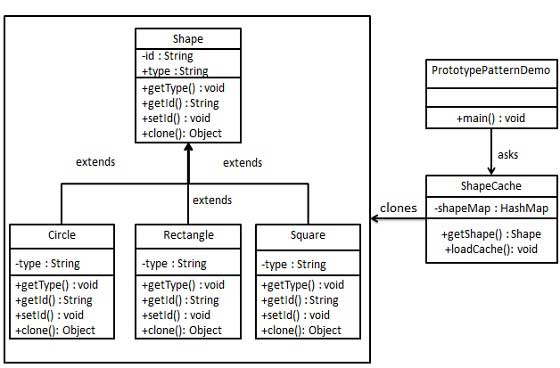# 原型模式

## 实现

PrototypePatternDemo，我们的演示类使用 ShapeCache 类来获取 Shape 对象。### 步骤 1

```public abstract class Shape implements Cloneable {

private String id;
protected String type;

abstract void draw();

public String getType(){
return type;
}

public String getId() {
return id;
}

public void setId(String id) {
this.id = id;
}

public Object clone() {
Object clone = null;
try {
clone = super.clone();
} catch (CloneNotSupportedException e) {
e.printStackTrace();
}
return clone;
}
}```

### 步骤 2

```public class Rectangle extends Shape {

public Rectangle(){
type = "Rectangle";
}

@Override
public void draw() {
System.out.println("Inside Rectangle::draw() method.");
}
}```
```public class Square extends Shape {

public Square(){
type = "Square";
}

@Override
public void draw() {
System.out.println("Inside Square::draw() method.");
}
}```
```public class Circle extends Shape {

public Circle(){
type = "Circle";
}

@Override
public void draw() {
System.out.println("Inside Circle::draw() method.");
}
}```

### 步骤 3

```import java.util.Hashtable;

public class ShapeCache {

private static Hashtable<String, Shape> shapeMap
= new Hashtable<String, Shape>();

public static Shape getShape(String shapeId) {
Shape cachedShape = shapeMap.get(shapeId);
return (Shape) cachedShape.clone();
}

// 对每种形状都运行数据库查询，并创建该形状
// shapeMap.put(shapeKey, shape);
// 例如，我们要添加三种形状
Circle circle = new Circle();
circle.setId("1");
shapeMap.put(circle.getId(),circle);

Square square = new Square();
square.setId("2");
shapeMap.put(square.getId(),square);

Rectangle rectangle = new Rectangle();
rectangle.setId("3");
shapeMap.put(rectangle.getId(),rectangle);
}
}```

### 步骤 4

PrototypePatternDemo 使用 ShapeCache 类来获取存储在 Hashtable 中的形状的克隆。

```public class PrototypePatternDemo {
public static void main(String[] args) {

Shape clonedShape = (Shape) ShapeCache.getShape("1");
System.out.println("Shape : " + clonedShape.getType());

Shape clonedShape2 = (Shape) ShapeCache.getShape("2");
System.out.println("Shape : " + clonedShape2.getType());

Shape clonedShape3 = (Shape) ShapeCache.getShape("3");
System.out.println("Shape : " + clonedShape3.getType());
}
}```

### 步骤 5

```Shape : Circle
Shape : Square
Shape : Rectangle```